Project

# Ambient Light Monitor: Display Measurements on an LCD

July 26, 2015 by Robert Keim

## Learn how to use an EFM8 microcontroller to convert current and voltage measurements to digits that can be displayed on an LCD. We'll discuss a convenient way to report analog-to-digital conversion values that represent current and voltage amplitudes.

Part 1 in the "How to Make an Ambient Light Monitor" Series

#### Recommended Level

Beginner/Intermediate

#### Project Overview

The overall objective of this project series is to design a smart ambient light monitor that can analyze indoor light levels and implement corresponding responsive actions, such as controlling a lamp dimmer. In the course of developing this project, we will need a convenient way to report analog-to-digital conversion values that represent current and voltage amplitudes. Thus, we will begin by writing firmware that can take an ordinary number stored in a variable and convert it into a series of individual digits, and then these digits will determine which arrays of pixel data we transfer to the LCD module.

This firmware is designed to display three-digit measurements with units of millivolts, volts, microamps, or milliamps. A decimal point following the first digit is automatically enabled if the displayed unit is volts or milliamps. This means that the display interface can process current amplitudes from 0 μA to 9.99 mA and voltage amplitudes from 0 mV to 9.99 V. Much of this range, though, will never be used—the maximum output current from the light sensor is 5 mA, and the ADC cannot measure voltages higher than its reference voltage, which in this design is 2.4 V.

#### Port I/O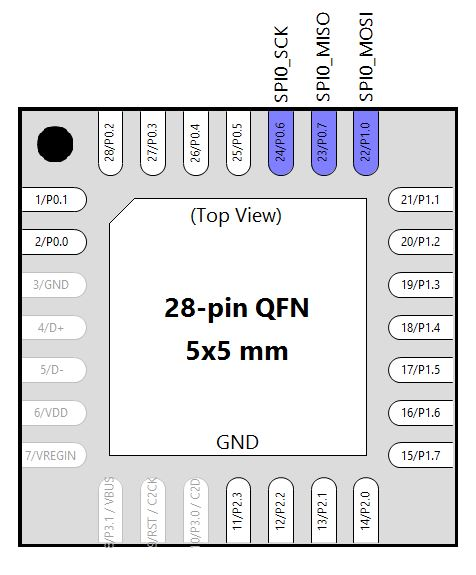You can refer to this article for more in-depth information about using the crossbar and configuring pins as inputs or outputs. As indicated by the above diagram, the SPI signals are enabled and routed to the pins that are connected to the corresponding LCD signals. The SPI chip select signal is controlled by firmware and output to P0.1 because the built-in SPI slave select signal is not compatible with the LCD interface.

#### Peripherals and Interrupts

At this stage in the project we need only two peripherals: SPI and Timer4. The SPI is configured for 3-wire master mode, and the clock divider is set so as to produce an SPI clock frequency of 875 kHz.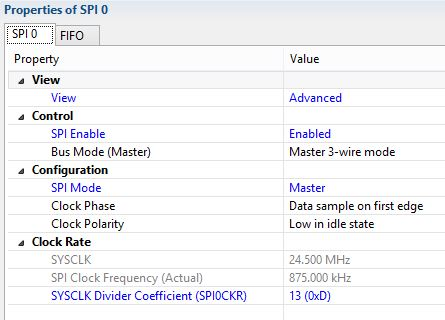The SPI interrupt is enabled because SPI transfers are governed by a state machine in the SPI interrupt service routine. The interrupt fires after each byte is transmitted. Timer4 is used for short delays, such as the setup and hold delays specified in the datasheet for the LCD module. One Timer4 count is about 490 ns, so if we need a delay of 6 μs, we set the Timer4 register to zero and wait until the count reaches 12.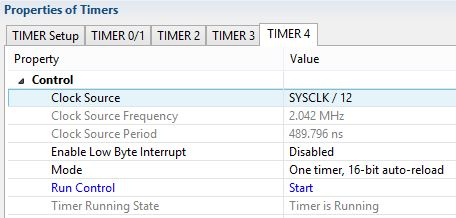#### Firmware

The firmware for this project can be divided into three main sections: the LCD communications interface, the function that converts a number stored in a variable into a series of individual digits, and the routines for updating the LCD pixel data array.

#### LCD Interface

We communicate with the LCD using multiple-line update mode, as described in a previous article. When the microcontroller boots up, it clears the LCD to all white pixels. The LCD is subsequently updated by writing 128 bits of pixel data to one or more line addresses. All LCD updates are initiated by the UpdateLCD() function in the “LCDControl.c” source file, and the data transfer process continues in the SPI interrupt service routine. The LCD communications interface in this project includes one improvement over what we used in previous articles: each call to UpdateLCD() can specify which portion of the display to update by putting the appropriate first and last line address into the LCDLineStart and LCDLineStop variables.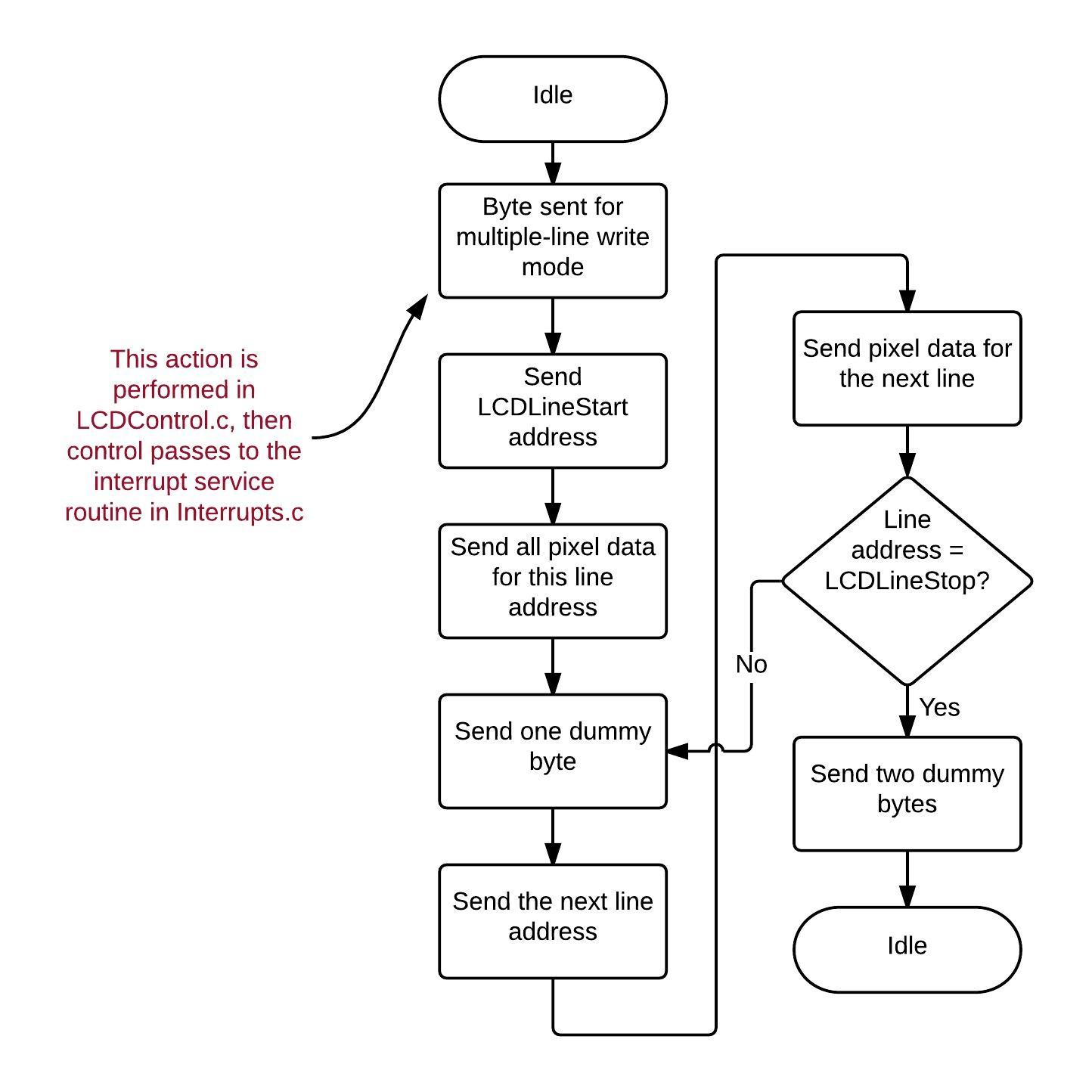#### Converting Numbers to Digits

It is important to understand that a numerical value stored in a variable is fundamentally different from a series of digits whereby we visualize a numerical value. A variable is simply a sequence of ones and zeros; this sequence can be interpreted in a variety of ways—for example, as an unsigned integer, a signed integer, or a floating-point value. Then further conversion is needed to express this interpreted value in a visual form. The standard C-language way to convert a variable value to a series of digits or characters is the printf() function, which is included in the library. But it is wise to avoid library routines when possible, primarily because designing your own code is more interesting, more rewarding, and more edifying. There are practical benefits as well, though, because your custom-designed code may provide all the desired functionality while also increasing execution speed or reducing memory requirements.

The key to the numerical conversion process is the modulus operator, represented by the “%” symbol:

/*the modulus operator is used to obtain the first digit, which
corresponds to the remainder that would result from dividing
by 10; we then twice divide the measured value by 10 and repeat
the modulus operation to obtain the remainders corresponding
to the next two digits*/
remainder = MeasuredValue % 10;
SetLCDDigit(DIGIT_POS_3, MatchDigittoArray(remainder));

MeasuredValue = MeasuredValue/10;
remainder = MeasuredValue % 10;
SetLCDDigit(DIGIT_POS_2, MatchDigittoArray(remainder));

MeasuredValue = MeasuredValue/10;
remainder = MeasuredValue % 10;
SetLCDDigit(DIGIT_POS_1, MatchDigittoArray(remainder));

The modulus operator returns the remainder that would result if you divided the variable’s integer value by the number on the right side of the “%” symbol. As shown in the code excerpt, we are using “MeasuredValue % 10” to extract the number corresponding to the rightmost digit. You can visualize this as shifting all the digits one place to the right and then lopping off the rightmost digit as it crosses the decimal point.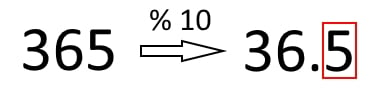Note, however, that the modulus operator does not actually alter the original value. So after extracting the first digit, we divide the original value by 10 and repeat the modulus operation to extract the next digit. The MatchDigittoArray() function contains a simple switch statement that determines which LCD pixel data array corresponds to the number contained in the remainder variable.

From Digit to Pixel Data

A two-dimensional array is used to hold LCD pixel data. In this project the pixel data array has 30 rows, because the digits are displayed in the middle 30 lines of the LCD screen. The following function copies the digit’s pixel values into the LCD pixel data array; the parameters passed to this function are the digit position (first, second, or third) and a pointer to the digit’s pixel data array. Similar code is used for displaying the appropriate unit abbreviation (μA, mA, mV, or V).

void SetLCDDigit(unsigned char DigitPosition, unsigned char *LCD_Digit)
{
unsigned char row;
unsigned char column_byte;
unsigned char column_byte_begin, column_byte_end;

/*this switch statement determines which column bytes to
modify based on the chosen digit position (first, second, or third)*/
switch(DigitPosition)
{
case DIGIT_POS_1: column_byte_begin = 0;
break;

case DIGIT_POS_2: column_byte_begin = DIGIT_WIDTH_BYTE;
break;

case DIGIT_POS_3: column_byte_begin = DIGIT_WIDTH_BYTE*2;
break;
}

column_byte_end = column_byte_begin + DIGIT_WIDTH_BYTE;

/*here the LCD display data array is loaded with the bytes
from the appropriate pixel data array generated by Scilab*/
for(row = 0; row < DIGIT_HEIGHT_PIX; row++)
{
for(column_byte = column_byte_begin; column_byte < column_byte_end; column_byte++)
{
LCDDisplayData[row][column_byte] = *LCD_Digit;
LCD_Digit++;
}
}

//wait until the SPI state variable indicates that the bus is available for a new transfer
while(LCDTxState != IDLE);

//the SPI state machine needs to know the first and last lines to be updated
LCDLineStart = DIGIT_BEGIN_LINE;
LCDLineStop = DIGIT_BEGIN_LINE + DIGIT_HEIGHT_PIX;
UpdateLCD();
}

The decimal point is automatically turned on if the unit is milliamps or volts, and it is automatically turned off if the unit is microamps or millivolts. The pixel data array is upated to display or not display the decimal point as follows:

void SetDecimalPoint(unsigned char DecimalPointStatus)
{
unsigned char row;
unsigned char column_byte;

//the decimal point can only be located after the first digit
column_byte = DIGIT_WIDTH_BYTE - 1;

/*the decimal point requires an area of 4 pixels by 4 pixels,
but the displayed shape is rounded because the top and bottom
lines have 2 horizontal black pixels and the 2 middle lines have
4 horizontal black pixels*/

/*note the use of bitwise AND and OR operations here: bitwise
operations are needed because the decimal point does not cover
8 horizontal pixels (i.e., one horizontal byte), and AND and OR are used
to ensure that other pixels in the byte are not altered*/

if(DecimalPointStatus == DEC_POINT_OFF)
{
row = (DIGIT_HEIGHT_PIX - DEC_POINT_HEIGHT);
LCDDisplayData[row][column_byte] |= BIT0;
LCDDisplayData[row][column_byte + 1]  |= BIT7;
row++;
LCDDisplayData[row][column_byte] |= (BIT1|BIT0);
LCDDisplayData[row][column_byte + 1] |= (BIT7|BIT6);
row++;
LCDDisplayData[row][column_byte] |= (BIT1|BIT0);
LCDDisplayData[row][column_byte + 1]  |= (BIT7|BIT6);
row++;
LCDDisplayData[row][column_byte] |= BIT0;
LCDDisplayData[row][column_byte + 1]  |= BIT7;
}

if(DecimalPointStatus == DEC_POINT_ON)
{
row = (DIGIT_HEIGHT_PIX - DEC_POINT_HEIGHT);
LCDDisplayData[row][column_byte] &= ~BIT0;
LCDDisplayData[row][column_byte + 1]  &= ~BIT7;
row++;
LCDDisplayData[row][column_byte] &= ~(BIT1|BIT0);
LCDDisplayData[row][column_byte + 1] &= ~(BIT7|BIT6);
row++;
LCDDisplayData[row][column_byte] &= ~(BIT1|BIT0);
LCDDisplayData[row][column_byte + 1] &= ~(BIT7|BIT6);
row++;
LCDDisplayData[row][column_byte] &= ~BIT0;
LCDDisplayData[row][column_byte + 1]  &= ~BIT7;
}

//wait until the SPI state variable indicates that the bus is available for a new transfer
while(LCDTxState != IDLE);

//the SPI state machine needs to know the first and last lines to be updated
LCDLineStart = DEC_PNT_LINE_BEGIN;
LCDLineStop = DEC_PNT_LINE_END;
UpdateLCD();
}

AmbientLightMonitor.zip

The while loop in “AmbientLightMonitor_main.c” looks like this:

number = 800;

while (1)
{
ConvertMeasurementandDisplay(CURRENT, number);

//these instructions provide a delay, so that the displayed number increments more slowly
while(LCDTxState != IDLE);
for(n = 0; n < 0xFFFF; n++)
SFRPAGE = TIMER4_PAGE; TMR4 = 0; while(TMR4 < 0xFF00);

number++;
if(number == 10000)	//ConvertMeasurementandDisplay() only accepts numbers up to 9999
number = 0;
}  

Digits_to_PixelData.zip

As shown below in the video, this will cause a continuously increasing measurement to appear on the LCD.

Scilab

The pixel data arrays for the digits and unit abbreviations were generated using a Scilab script that incorporates functionality discussed in two previous articles (this one and this one). In this project, the digits (and thus also the digit .bmp images processed with this script) have dimensions of 30 vertical pixels by 24 horizontal pixels. These are much larger and more visually appealing than 10-by-8-pixel characters used previously. Note that the horizontal dimension was again chosen as a multiple of 8 to ensure that we will not need to use awkward bitwise operations when updating the LCD pixel data array.

Next Article in Series: Ambient Light Monitor: Understanding and Implementing the ADC

Give this project a try for yourself! Get the BOM.